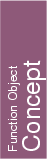## Kernel::BoundedSide_2

A model for this must provide:

Bounded_side
 fo.operator() ( Kernel::Circle_2 c, Kernel::Point_2 p)
returns either ON_UNBOUNDED_SIDE, ON_BOUNDED_SIDE, or the constant ON_BOUNDARY, depending on where point p is relative to circle c.

Bounded_side
 fo.operator() ( Kernel::Triangle_2 t, Kernel::Point_2 p)
returns either ON_UNBOUNDED_SIDE, ON_BOUNDED_SIDE, or the constant ON_BOUNDARY, depending on where point p is relative to triangle t.

Bounded_side
 fo.operator() ( Kernel::Iso_rectangle_2 r, Kernel::Point_2 p)
returns either ON_UNBOUNDED_SIDE, ON_BOUNDED_SIDE, or the constant ON_BOUNDARY, depending on where point p is relative to rectangle r.

### Refines

AdaptableFunctor (with two arguments)

### See Also

CGAL::Circle_2<Kernel>
CGAL::Triangle_2<Kernel>
CGAL::Iso_rectangle_2<Kernel>